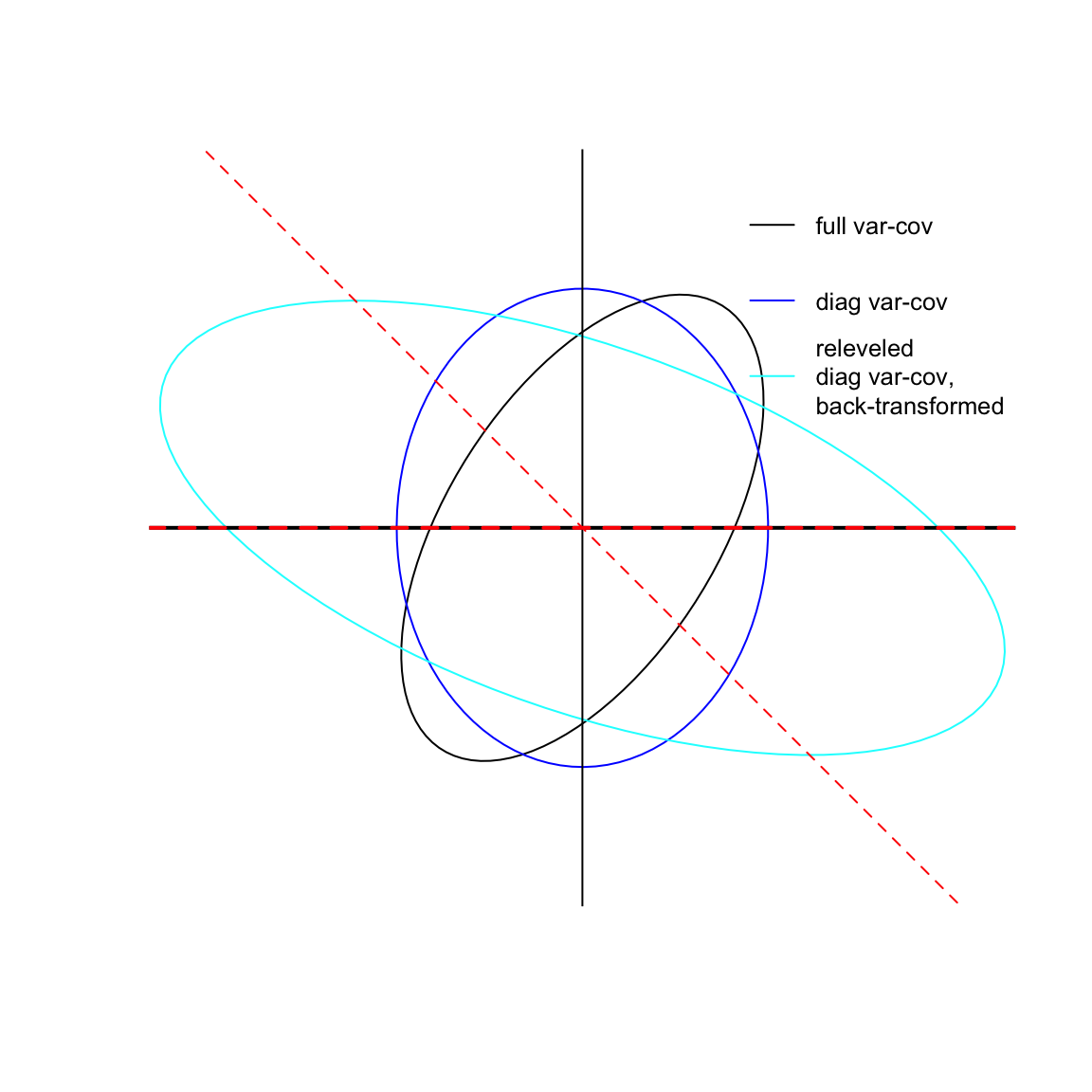We normally have an expectation, derived from fitting standard linear models, that making linear transformations of the predictor variables (e.g., centering continuous variables, releveling factors, or changing contrasts) will have effects on the estimated parameters and associated hypothesis tests, but not on the overall model. We will get the same overall log-likelihood, and can reconstruct the original parameters if we need to.

For example:

set.seed(101)
dd <- data.frame(x=1:10,y=rnorm(10))
dd$cx <- dd$x-mean(dd$x) m1 <- lm(y~x,data=dd) m2 <- lm(y~cx,data=dd) Same log-likelihood, same coefficients once we adjust the intercept: all.equal(logLik(m1),logLik(m2)) ##  TRUE slope <- coef(m2) all.equal(coef(m1),coef(m2)+c(-slope*mean(dd$x),0),
check.attributes=FALSE)
##  TRUE

In general, if we want to translate parameters according to some linear transformation matrix $$\boldsymbol C$$ (i.e., each row of the matrix defines a new parameter in terms of linear combinations of the old parameters), $$\beta' = \boldsymbol C \beta$$, then the variance-covariance matrix is translated according to $$\boldsymbol C \boldsymbol V \boldsymbol C^\top$$.

Example from Roger Mundry:

library(lme4)

Generate data (hidden function), a balanced 10x10 design (10 subjects, 10 observations within subject, evenly split between two treatments).

dd <- mkData(seed=39)
with(dd,table(within.fac,rfac))
##           rfac
## within.fac 1 2 3 4 5 6 7 8 9 10
##          a 5 5 5 5 5 5 5 5 5  5
##          b 5 5 5 5 5 5 5 5 5  5

Fit models with and without covariance among random-effects terms, and with original and releveled factors:

nodiag1 <- lmer(resp~within.fac+(1+with.fac.code||rfac), REML=FALSE, data=dd)
nodiag2 <- update(nodiag1,.~rel.within.fac+(1+rel.with.fac.code||rfac))
full1   <- update(nodiag1,.~within.fac    +(1+    with.fac.code|rfac))
full2   <- update(nodiag1,.~rel.within.fac+(1+rel.with.fac.code|rfac))
all.equal(logLik(full1),logLik(full2))
##  TRUE
all.equal(logLik(nodiag1),logLik(nodiag2))
##  "Mean relative difference: 0.03857462"

If we use the default coding (treatment level a first), we have $$\beta_0$$ equal to the (conditional) mean of group a, $$\beta_1$$ equal to the difference (b-a, as it were). If we want to switch to the other coding, we need $$\beta'_0 = \beta_0+\beta_1$$, $$\beta'_1 = -\beta_1$$, so we have a transformation matrix $$\boldsymbol C = \begin{pmatrix} 1 & 1 \\ 0 & -1 \end{pmatrix}$$.

tmat <- matrix(c(1,1,0,-1),2,byrow=TRUE)
## compare fixed-effect parameters
eqfun(tmat %*% fixef(full1), fixef(full2))
##  TRUE
## compare random-effects variance-covariance matrices
v1 <- VarCorr(full1)[]
v2 <- VarCorr(full2)[]
eqfun(tmat %*% v1 %*% t(tmat),v2,tolerance=1e-6)
##  TRUE
v3 <- diag(unlist(VarCorr(nodiag1)))
v4 <- diag(unlist(VarCorr(nodiag2)))
v5 <- tmat %*% v4 %*% t(tmat) ## back-transform to original scale